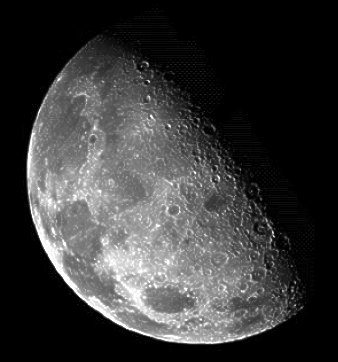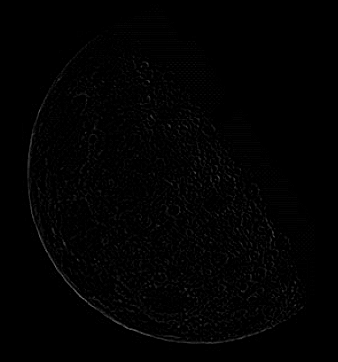While studying for my digital image processing final exam yesterday, I came back across unsharp masking. When I first saw this, I thought it was really neat. This time around, I took the hands-on approach and tried it myself in Octave. It has been used by the publishing and printing industry for years.

Unsharp masking is a method of sharpening an image. The idea is this,

1. Blur the original image.
2. Subtract the blurred image from the original, creating a mask.
3. Add the mask to the original image.

Here is an example using a 1-dimensional signal. I blurred the signal with a 1x5 averaging filter: `[1 1 1 1 1] * 1/5`. Then I subtracted the blurred signal from the original to create a mask. Finally, I added the unsharp mask to the original signal. For images, we do this in 2-dimensions, as an image is simply a 2-dimensional signal.When it comes to image processing, we can create the mask in one easy step! This is done by performing a 2-dimensional convolution with a Laplacian kernel. It does steps 1 and 2 at the same time. This is the Laplacian I used in the example at the beginning,So, to do it in Octave, this is all you need,

``````octave> i = imread("moon.png");
octave> m = conv2(i, [0 -1 0; -1 4 -1; 0 -1 0], "same");
octave> imwrite("moon-sharp.png", i + 2 * uint8(m))
``````

`i` is the image and `m` is the mask. The mask created in step 2 looks like this,You could take the above Octave code and drop it into a little she-bang script to create a simple image sharpening program. I leave this as an exercise for the reader.